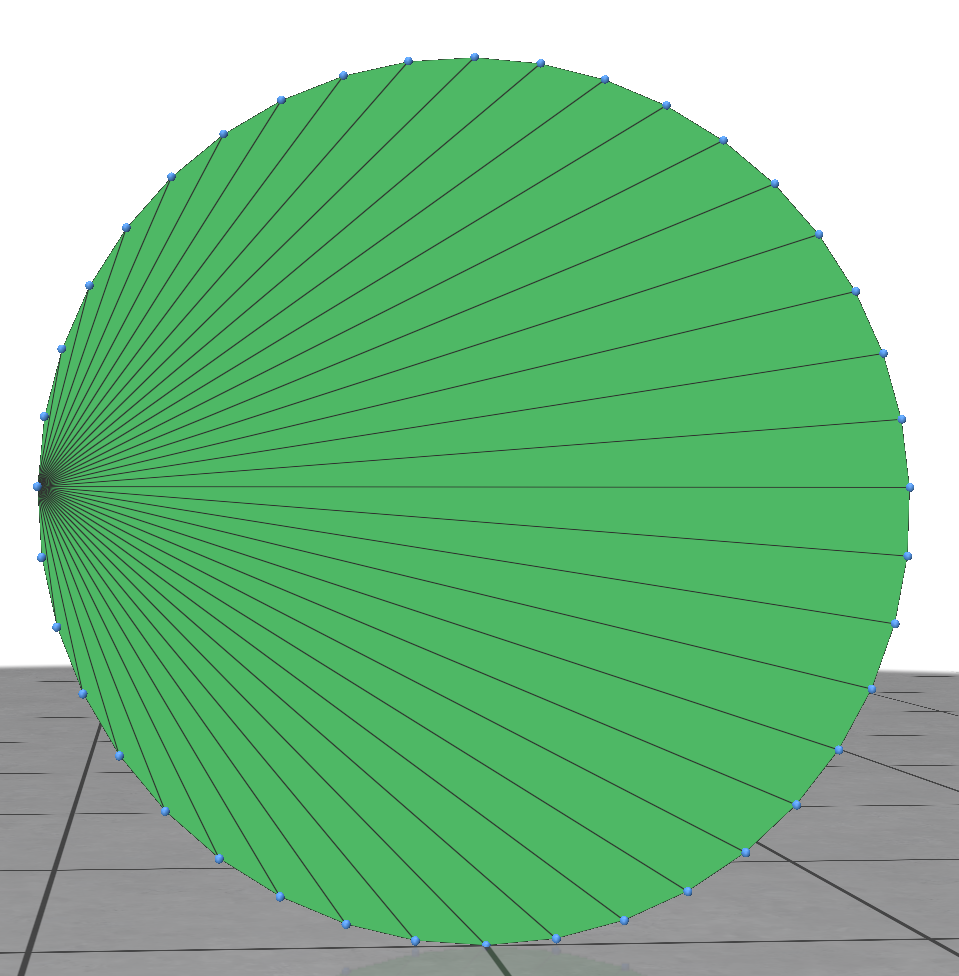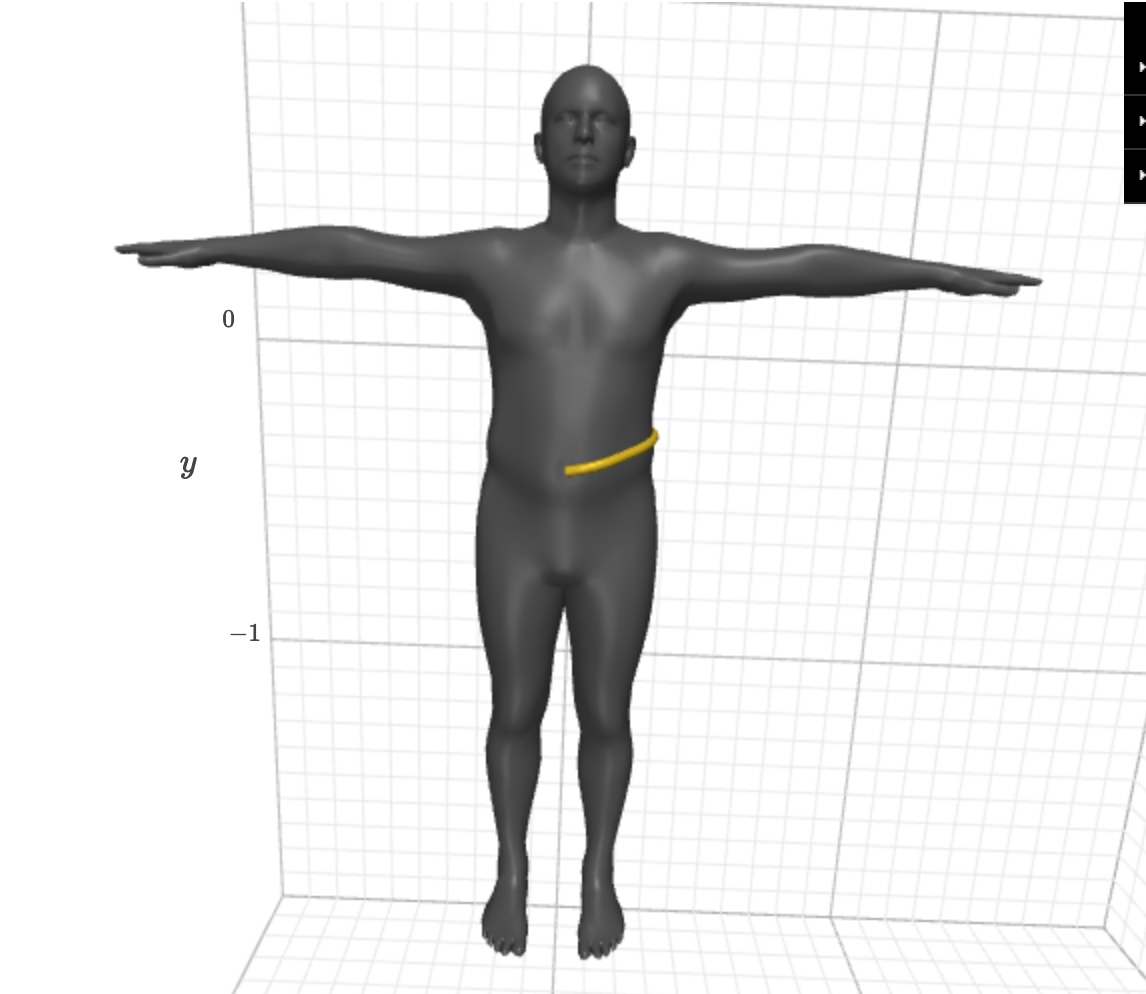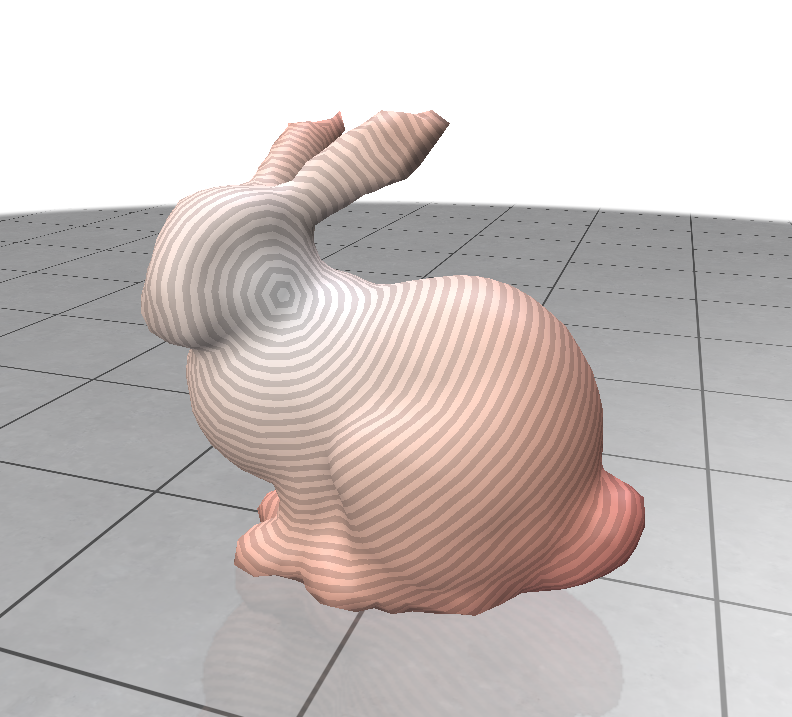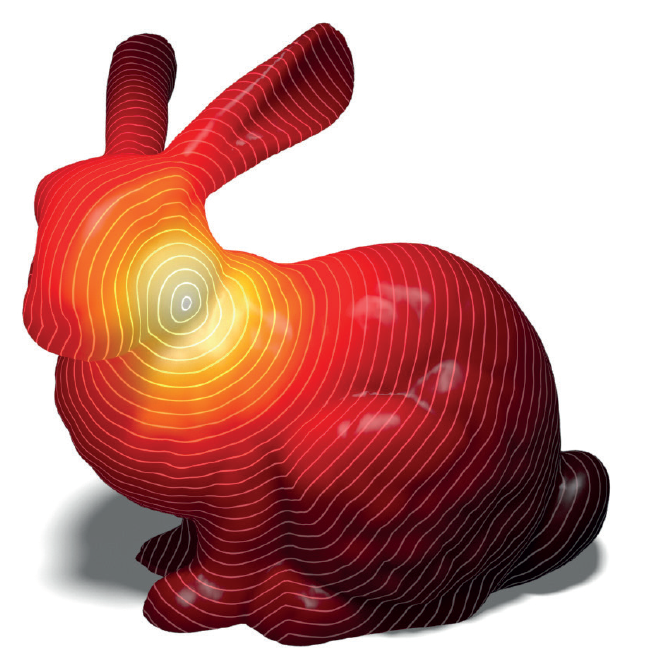potpourri3d

# potpourri3d

A Python library of various algorithms and utilities for 3D triangle meshes and point clouds. Managed by Nicholas Sharp, with new tools added lazily as needed. Currently, mainly bindings to C++ tools from geometry-central.

`pip install potpourri3d`

The blend includes:

• Mesh and point cloud reading/writing to a few file formats
• Use heat methods to compute distance, parallel transport, logarithmic maps, and more

## Installation

Potpourri3d is on the pypi package index with precompiled binaries for most configuations. Get it like:

`pip install potpourri3d`

If none of the precompiled binaries match your system, `pip` will attempt to compile the library from scratch. This requires `cmake` and a workng C++ compiler toolchain.

Note: Some bound functions invoke sparse linear solvers internally. The precompiled binaries use Eigen's solvers; using Suitesparse's solvers instead may significantly improve performance & robustness. To get them, locally compile the package on a machine with Suitesparse installed using the command below (relevant docs).

``````python -m pip install potpourri3d --no-binary potpourri3d
``````

## Documentation

### Input / Output

Read/write meshes and point clouds from some common formats.

• `read_mesh(filename)` Reads a mesh from file. Returns numpy matrices `V, F`, a Nx3 real numpy array of vertices and a Mx3 integer numpy array of 0-based face indices (or Mx4 for a quad mesh, etc).

• `filename` the path to read the file from. Currently supports the same file types as geometry-central. The file type is inferred automatically from the path extension.
• `write_mesh(V, F, filename)` Write a mesh from file. Returns numpy matrices `V, F`, a Vx3 real array of vertices and a Fx3 integer array of 0-based face indices (or Fx4 for a quad mesh, etc).

• `V` a Nx3 real numpy array of vertices
• `F` a Mx3 integer numpy array of faces, with 0-based vertex indices (or Mx4 for a quad mesh, etc).
• `filename` the path to write the file to. Currently supports the same file types as geometry-central. The file type is inferred automatically from the path extension.

### Mesh Distance

Use the heat method for geodesic distance to compute geodesic distance on surfaces. Repeated solves are fast after initial setup. Uses intrinsic triangulations internally for increased robustness.

```import potpourri3d as pp3d

# = Stateful solves (much faster if computing distance many times)
solver = pp3d.MeshHeatMethodDistanceSolver(V,F)
dist = solver.compute_distance(7)
dist = solver.compute_distance_multisource([1,2,3])

# = One-off versions
dist = pp3d.compute_distance(V,F,7)
dist = pp3d.compute_distance_multisource(V,F,[1,3,4])```
• `MeshHeatMethodDistanceSolver(self, V, F, t_coef=1., use_robust=True)` construct an instance of the solver class.
• `V` a Nx3 real numpy array of vertices
• `F` a Mx3 integer numpy array of faces, with 0-based vertex indices (triangle meshes only, but need not be manifold).
• `t_coef` set the time used for short-time heat flow. Generally don't change this. If necessary, larger values may make the solution more stable at the cost of smoothing it out.
• `use_robust` use intrinsic triangulations for increased robustness. Generaly leave this enabled.
• `MeshHeatMethodDistanceSolver.compute_distance(v_ind)` compute distance from a single vertex, given by zero-based index. Returns an array of distances.
• `MeshHeatMethodDistanceSolver.compute_distance_multisource(v_ind_list)` compute distance from the nearest of a collection of vertices, given by a list of zero-based indices. Returns an array of distances.
• `compute_distance(V, F, v_ind)` Similar to above, but one-off instead of stateful. Returns an array of distances.
• `compute_distance_multisource(V, F, v_ind_list)` Similar to above, but one-off instead of stateful. Returns an array of distances.

### Mesh Vector Heat

Use the vector heat method to compute various interpolation & vector-based quantities on meshes. Repeated solves are fast after initial setup.

```import potpourri3d as pp3d

# = Stateful solves
V, F = # a Nx3 numpy array of points and Mx3 array of triangle face indices
solver = pp3d.MeshVectorHeatSolver(V,F)

# Extend the value `0.` from vertex 12 and `1.` from vertex 17. Any vertex
# geodesically closer to 12. will take the value 0., and vice versa
# (plus some slight smoothing)
ext = solver.extend_scalar([12, 17], [0.,1.])

# Get the tangent frames which are used by the solver to define tangent data
# at each vertex
basisX, basisY, basisN = solver.get_tangent_frames()

# Parallel transport a vector along the surface
# (and map it to a vector in 3D)
sourceV = 22
ext = solver.transport_tangent_vector(sourceV, [6., 6.])
ext3D = ext[:,0,np.newaxis] * basisX +  ext[:,1,np.newaxis] * basisY

# Compute the logarithmic map
logmap = solver.compute_log_map(sourceV)
• `MeshVectorHeatSolver(self, V, F, t_coef=1.)` construct an instance of the solver class.
• `V` a Nx3 real numpy array of vertices
• `F` a Mx3 integer numpy array of faces, with 0-based vertex indices (triangle meshes only, should be manifold).
• `t_coef` set the time used for short-time heat flow. Generally don't change this. If necessary, larger values may make the solution more stable at the cost of smoothing it out.
• `MeshVectorHeatSolver.extend_scalar(v_inds, values)` nearest-geodesic-neighbor interpolate values defined at vertices. Vertices will take the value from the closest source vertex (plus some slight smoothing)
• `v_inds` a list of source vertices
• `values` a list of scalar values, one for each source vertex
• `MeshVectorHeatSolver.get_tangent_frames()` get the coordinate frames used to define tangent data at each vertex. Returned as a tuple of basis-X, basis-Y, and normal axes, each as an Nx3 array. May be necessary for change-of-basis into or out of tangent vector convention.
• `MeshVectorHeatSolver.transport_tangent_vector(v_ind, vector)` parallel transports a single vector across a surface
• `v_ind` index of the source vertex
• `vector` a 2D tangent vector to transport
• `MeshVectorHeatSolver.transport_tangent_vectors(v_inds, vectors)` parallel transports a collection of vectors across a surface, such that each vertex takes the vector from its nearest-geodesic-neighbor.
• `v_inds` a list of source vertices
• `vectors` a list of 2D tangent vectors, one for each source vertex
• `MeshVectorHeatSolver.compute_log_map(v_ind)` compute the logarithmic map centered at the given source vertex
• `v_ind` index of the source vertex

### Point Cloud Distance & Vector Heat

Use the heat method for geodesic distance and vector heat method to compute various interpolation & vector-based quantities on point clouds. Repeated solves are fast after initial setup.```import potpourri3d as pp3d

# = Stateful solves
P = # a Nx3 numpy array of points
solver = pp3d.PointCloudHeatSolver(P)

# Compute the geodesic distance to point 4
dists = solver.compute_distance(4)

# Extend the value `0.` from point 12 and `1.` from point 17. Any point
# geodesically closer to 12. will take the value 0., and vice versa
# (plus some slight smoothing)
ext = solver.extend_scalar([12, 17], [0.,1.])

# Get the tangent frames which are used by the solver to define tangent data
# at each point
basisX, basisY, basisN = solver.get_tangent_frames()

# Parallel transport a vector along the surface
# (and map it to a vector in 3D)
sourceP = 22
ext = solver.transport_tangent_vector(sourceP, [6., 6.])
ext3D = ext[:,0,np.newaxis] * basisX +  ext[:,1,np.newaxis] * basisY

# Compute the logarithmic map
logmap = solver.compute_log_map(sourceP)```
• `PointCloudHeatSolver(self, P, t_coef=1.)` construct an instance of the solver class.
• `P` a Nx3 real numpy array of points
• `t_coef` set the time used for short-time heat flow. Generally don't change this. If necessary, larger values may make the solution more stable at the cost of smoothing it out.
• `PointCloudHeatSolver.extend_scalar(p_inds, values)` nearest-geodesic-neighbor interpolate values defined at points. Points will take the value from the closest source point (plus some slight smoothing)
• `v_inds` a list of source points
• `values` a list of scalar values, one for each source points
• `PointCloudHeatSolver.get_tangent_frames()` get the coordinate frames used to define tangent data at each point. Returned as a tuple of basis-X, basis-Y, and normal axes, each as an Nx3 array. May be necessary for change-of-basis into or out of tangent vector convention.
• `PointCloudHeatSolver.transport_tangent_vector(p_ind, vector)` parallel transports a single vector across a surface
• `p_ind` index of the source point
• `vector` a 2D tangent vector to transport
• `PointCloudHeatSolver.transport_tangent_vectors(p_inds, vectors)` parallel transports a collection of vectors across a surface, such that each vertex takes the vector from its nearest-geodesic-neighbor.
• `p_inds` a list of source points
• `vectors` a list of 2D tangent vectors, one for each source point
• `PointCloudHeatSolver.compute_log_map(p_ind)` compute the logarithmic map centered at the given source point
• `p_ind` index of the source point
• #### Randomly generated mesh test fails

Hello!

I've been trying to figure out how to generate a 2D circle mesh for use with `potpourri3d`, but I keep getting this error: `self-edge in face list [x] -- [x]`. While investigating, I came across your test functions, `generate_verts()` and `generate_faces()`. When I tried to load a mesh using those two, I also got `self-edge in face list`. However, puzzlingly, I could use the bunny mesh without any errors.

Other errors I got while trying to make my circle mesh work include `vertex [x] appears in more than one boundary loop` and `duplicate edge in list [i] -- [j]`.

I'm confused because `polyscope` renders the meshes correctly, but `potpourri3d` has a hard time with the same meshes.

Thank you!``````import numpy as np
import polyscope as ps
import potpourri3d as pp3d

# Initialize polyscope
ps.init()

def generate_verts(n_pts=999):
np.random.seed(777)
return np.random.rand(n_pts, 3)

def generate_faces(n_pts=999):
# n_pts should be a multiple of 3 for indexing to work out
np.random.seed(777)
rand_faces = np.random.randint(0, n_pts, size=(2*n_pts,3))
coverage_faces = np.arange(n_pts).reshape(-1, 3)
faces = np.vstack((rand_faces, coverage_faces))
return faces

verts = generate_verts()
faces = generate_faces()
solver = pp3d.MeshVectorHeatSolver(verts, faces)

solver = pp3d.MeshVectorHeatSolver(verts, faces)

verts = unit_circle
faces = []
for i in range(0, verts.shape):
if i == verts.shape-1:
faces.append([i, 0, 0])
else:
faces.append([i, i+1, 0])
faces = np.array(faces)
solver = pp3d.MeshVectorHeatSolver(verts, faces)
ps.register_surface_mesh("unit circle mesh", verts, faces, smooth_shade=True)

ps.show()
``````
opened by mhr 2
• #### Geodesic from a predefined path?

Thank you so much for making the python binding! I use mainly use python for geometry stuff, so this is extremely helpful.

I'm working on some anthropometry, and trying to extract the rise curve, from the navel down the crotch and up to the lower back. I'm trying to use the edge flip solver for this, but the shorter path it found is around the side of the torso. According to the paper, it seems it should be possible to optimize a path through a different direction. Would that be possible to do with the python binding?enhancement
opened by panangam 2
• #### RuntimeError: vertices lie on disconnected components of the surface

I load a mesh with triangle faces. Then I try to execute these code lines: ` path_solver = pp3d.EdgeFlipGeodesicSolver(V, F) # shares precomputation for repeated solves

path_pts = path_solver.find_geodesic_path(v_start=0, v_end=100)`

But launch "RuntimeError: vertices lie on disconnected components of the surface".

How to solve this problem??

opened by facundolazcano 2
• #### Poor geodesic distance accuracy in a simple case

Forgive me if my expectations for accuracy are unreasonable for the HEAT method. I have the following minimal example

``````import numpy as np
from potpourri3d import MeshHeatMethodDistanceSolver

solver = MeshHeatMethodDistanceSolver(np.array([[0, 0, 0], [1, 0, 0], [0, 1, 0]]), np.array([[0, 1, 2]]))
solver.compute_distance(0) # -> array([0.        , 0.70710654, 0.70710654])
solver.compute_distance(1) # -> array([0.91430181, 0.        , 1.31933315])
solver.compute_distance(2) # -> array([0.91430181, 1.31933315, 0.        ])
``````

The fact that the first example is returning distances of `sqrt(2)/2` makes it seem like this is a bug rather than a limitation of the method somehow.

Its worth noting that setting `t_coef=0.001` makes the results of the second two cases more accurate, but the first case returns `[0. , 0.70710654, 0.70710654]` no matter the value of `t_coef`

opened by brucedjones 0
• #### Can the position coordinates of the contour line be obtained？At present, the isoline distance field generated by potpourri3d and polyscope cannot achieve the same effect as in the paper, and the isoline coordinates cannot be obtained. Can this problem be fixed or suggestions for the algorithm of the isoline?

opened by cheng-c210 0

• #### v0.0.1(Feb 17, 2021)

###### Nicholas Sharp###### DeepFaceEditing: Deep Face Generation and Editing with Disentangled Geometry and Appearance Control

DeepFaceEditing: Deep Face Generation and Editing with Disentangled Geometry and Appearance Control One version of our system is implemented using the

197 Oct 19, 2021
###### Geometry-Free View Synthesis: Transformers and no 3D Priors

Geometry-Free View Synthesis: Transformers and no 3D Priors Geometry-Free View Synthesis: Transformers and no 3D Priors Robin Rombach*, Patrick Esser*

208 Oct 21, 2021
###### An implementation of the research paper "Retina Blood Vessel Segmentation Using A U-Net Based Convolutional Neural Network"

Retina Blood Vessels Segmentation This is an implementation of the research paper "Retina Blood Vessel Segmentation Using A U-Net Based Convolutional

20 Oct 8, 2021
###### Based on the paper "Geometry-aware Instance-reweighted Adversarial Training" ICLR 2021 oral

Geometry-aware Instance-reweighted Adversarial Training This repository provides codes for Geometry-aware Instance-reweighted Adversarial Training (ht

34 Sep 8, 2021
###### Multiview Neural Surface Reconstruction by Disentangling Geometry and Appearance

Multiview Neural Surface Reconstruction by Disentangling Geometry and Appearance Project Page | Paper | Data This repository contains an implementatio

277 Oct 22, 2021
###### Data and Code for ACL 2021 Paper "Inter-GPS: Interpretable Geometry Problem Solving with Formal Language and Symbolic Reasoning"

Introduction Code and data for ACL 2021 Paper "Inter-GPS: Interpretable Geometry Problem Solving with Formal Language and Symbolic Reasoning". We cons

54 Sep 28, 2021
###### 🛠 All-in-one web-based IDE specialized for machine learning and data science.

All-in-one web-based development environment for machine learning Getting Started • Features & Screenshots • Support • Report a Bug • FAQ • Known Issu

2.2k Oct 23, 2021
###### Generate vibrant and detailed images using only text.

CLIP Guided Diffusion From RiversHaveWings. Generate vibrant and detailed images using only text. See captions and more generations in the Gallery See

112 Oct 15, 2021
###### Add-on for importing and auto setup of character creator 3 character exports.

CC3 Blender Tools An add-on for importing and automatically setting up materials for Character Creator 3 character exports. Using Blender in the Chara

91 Oct 21, 2021
###### image scene graph generation benchmark

Scene Graph Benchmark in PyTorch 1.7 This project is based on maskrcnn-benchmark Highlights Upgrad to pytorch 1.7 Multi-GPU training and inference Bat

153 Oct 20, 2021
###### Towers of Babel: Combining Images, Language, and 3D Geometry for Learning Multimodal Vision. ICCV 2021.

Towers of Babel: Combining Images, Language, and 3D Geometry for Learning Multimodal Vision Download links and PyTorch implementation of "Towers of Ba

35 Oct 8, 2021
###### Unofficial & improved implementation of NeRF--: Neural Radiance Fields Without Known Camera Parameters

[Unofficial code-base] NeRF--: Neural Radiance Fields Without Known Camera Parameters [ Project | Paper | Official code base ] ⬅️ Thanks the original

120 Oct 21, 2021
###### implicit displacement field

Geometry-Consistent Neural Shape Representation with Implicit Displacement Fields [project page][paper][cite] Geometry-Consistent Neural Shape Represe

44 Oct 1, 2021
###### Image transformations designed for Scene Text Recognition (STR) data augmentation. Published at ICCV 2021 Workshop on Interactive Labeling and Data Augmentation for Vision.

Data Augmentation for Scene Text Recognition (ICCV 2021 Workshop) (Pronounced as "strog") Paper Arxiv Why it matters? Scene Text Recognition (STR) req

59 Oct 18, 2021
###### [ICCV 2021 Oral] PoinTr: Diverse Point Cloud Completion with Geometry-Aware Transformers

PoinTr: Diverse Point Cloud Completion with Geometry-Aware Transformers Created by Xumin Yu*, Yongming Rao*, Ziyi Wang, Zuyan Liu, Jiwen Lu, Jie Zhou

127 Oct 15, 2021
###### Code for ECCV 2020 paper "Contacts and Human Dynamics from Monocular Video".

Contact and Human Dynamics from Monocular Video This is the official implementation for the ECCV 2020 spotlight paper by Davis Rempe, Leonidas J. Guib

112 Oct 18, 2021
###### Curvlearn, a Tensorflow based non-Euclidean deep learning framework.

English | 简体中文 Why Non-Euclidean Geometry Considering these simple graph structures shown below. Nodes with same color has 2-hop distance whereas 1-ho

64 Oct 13, 2021
###### Like ThreeJS but for Python and based on wgpu

pygfx A render engine, inspired by ThreeJS, but for Python and targeting Vulkan/Metal/DX12 (via wgpu). Introduction This is a Python render engine bui

33 Oct 17, 2021
###### Video Contrastive Learning with Global Context

Video Contrastive Learning with Global Context (VCLR) This is the official PyTorch implementation of our VCLR paper. Install dependencies environments

102 Oct 18, 2021